GeeksforGeeks App
Open AppBrowser
Continue

# Autism Prediction using Machine Learning

Autism is a neurological disorder that affects a person’s ability to interact with others, make eye contact with others, learn capacity, and other behavioral and social capabilities of a person.

But there is no ascertain way to tell whether a person has Autism or not because there are no such diagnostics methods available to diagnose this disorder. But what if we use machine learning to predict whether a person suffers from Autism or not? This is exactly what we’ll be learning in this article.

## Importing Libraries and Dataset

Python libraries make it very easy for us to handle the data and perform typical and complex tasks with a single line of code.

• Pandas – This library helps to load the data frame in a 2D array format and has multiple functions to perform analysis tasks in one go.
• Numpy – Numpy arrays are very fast and can perform large computations in a very short time.
• Matplotlib/Seaborn – This library is used to draw visualizations.
• Sklearn – This module contains multiple libraries having pre-implemented functions to perform tasks from data preprocessing to model development and evaluation.
• XGBoost – This contains the eXtreme Gradient Boosting machine learning algorithm which is one of the algorithms which helps us to achieve high accuracy on predictions.
• Imblearn – This module contains a function that can be used for handling problems related to data imbalance.

## Python3

 `import` `numpy as np``import` `pandas as pd``import` `matplotlib.pyplot as plt``import` `seaborn as sb``from` `sklearn.model_selection ``import` `train_test_split``from` `sklearn.preprocessing ``import` `LabelEncoder, StandardScaler``from` `sklearn ``import` `metrics``from` `sklearn.svm ``import` `SVC``from` `xgboost ``import` `XGBClassifier``from` `sklearn.linear_model ``import` `LogisticRegression``from` `imblearn.over_sampling ``import` `RandomOverSampler` `import` `warnings``warnings.filterwarnings(``'ignore'``)`

Now let’s load the dataset into the pandas data frame and print its first five rows.

## Python3

 `df ``=` `pd.read_csv(``'train.csv'``)``print``(df.head())`

Output: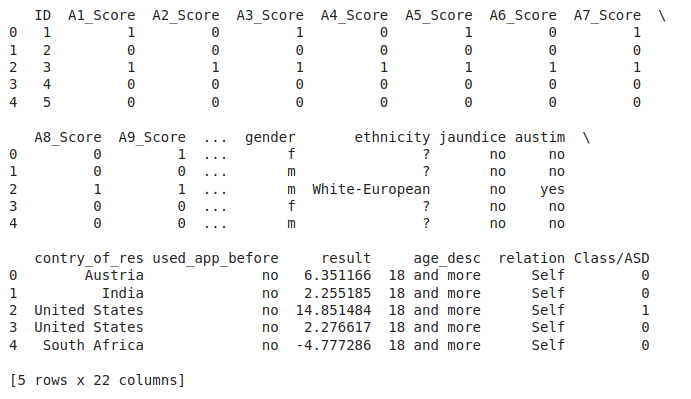First Five rows of the dataset

Now let’s check the size of the dataset.

## Python3

 `df.shape`

Output:

`(800, 22)`

Let’s check which column of the dataset contains which type of data.

## Python3

 `df.info()`

Output: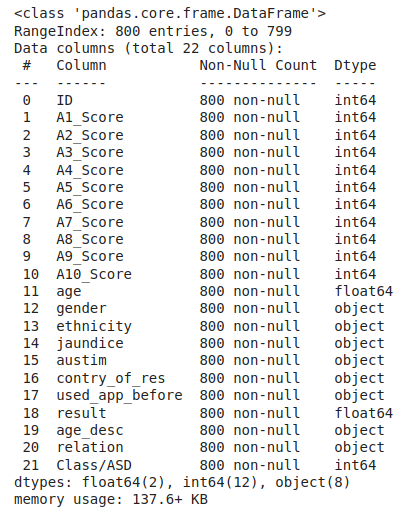Information regarding data in the columns

As per the above information regarding the data in each column we can observe that there are no null values.

## Python3

 `df.describe().T`

Output: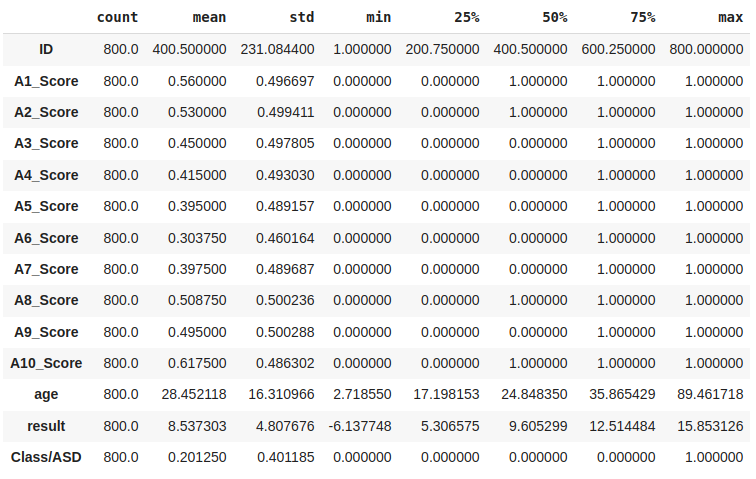Descriptive statistical measures of the dataset

## Data Cleaning

The data which is obtained from the primary sources is termed the raw data and required a lot of preprocessing before we can derive any conclusions from it or to some modeling on it. Those preprocessing steps are known as data cleaning and it includes, outliers removal, null value imputation, and removing discrepancies of any sort in the data inputs.

## Python3

 `df[``'ethnicity'``].value_counts()`

Output: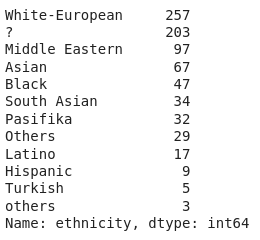value_count of each unique value in the column

In the above two outputs we can observe some ambiguity that there are ‘?’, ‘others’, and ‘Others’ which all must be the same as they are unknown or we can say that null values have been substituted with some indicator.

## Python3

 `df[``'relation'``].value_counts()`

Output: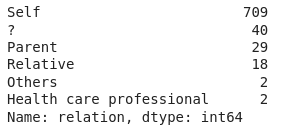value_count of each unique value in the column

The same is the case with this column so, let’s clean this data, and along with this let’s convert ‘yes’ and ‘no’ to 0 and 1.

## Python3

 `df ``=` `df.replace({``'yes'``:``1``, ``'no'``:``0``, ``'?'``:``'Others'``, ``'others'``:``'Others'``})`

Now we have cleaned the data a bit to derive insights from it.

## Exploratory Data Analysis

EDA is an approach to analyzing the data using visual techniques. It is used to discover trends, and patterns, or to check assumptions with the help of statistical summaries and graphical representations. Here we will see how to check the data imbalance and skewness of the data.

## Python3

 `plt.pie(df[``'Class/ASD'``].value_counts().values, autopct``=``'%1.1f%%'``)``plt.show()`

Output: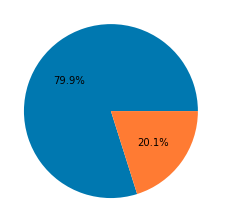Pie chart for the number of data for each target

The dataset we have is highly imbalanced. If we will train our model using this data then the model will face a hard time predicting the positive class which is our main objective here to predict whether a person has autism or not with high accuracy.

## Python3

 `ints ``=` `[]``objects ``=` `[]``floats ``=` `[]` `for` `col ``in` `df.columns:``  ``if` `df[col].dtype ``=``=` `int``:``    ``ints.append(col)``  ``elif` `df[col].dtype ``=``=` `object``:``    ``objects.append(col)``  ``else``:``    ``floats.append(col)`

Now, we have columns segregated based on the type of data they contain hence now we’ll be able to perform suitable analysis depending upon the type of data.

## Python3

 `ints.remove(``'ID'``)``ints.remove(``'Class/ASD'``)`

The ‘ID’ column will contain a unique value for each of the rows and for the column ‘Class/ASD’ we have already analyzed its distribution so, that is why they have been removed in the above code.

## Python3

 `plt.subplots(figsize``=``(``15``,``15``))` `for` `i, col ``in` `enumerate``(ints):``  ``plt.subplot(``4``,``3``,i``+``1``)``  ``sb.countplot(df[col], hue``=``df[``'Class/ASD'``])``plt.tight_layout()``plt.show()`

Output: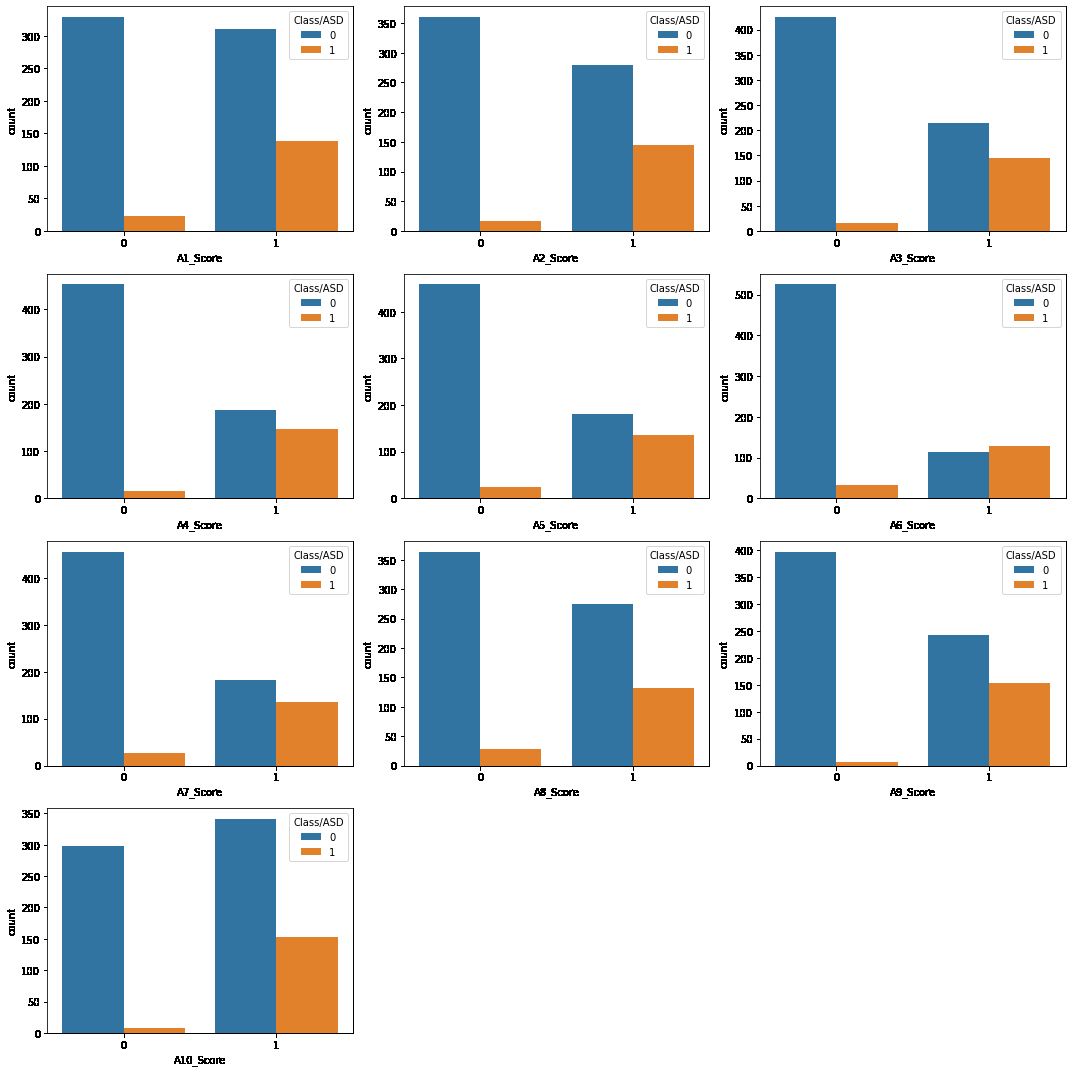Count plots for each of the numerical column

From these plots, we can conclude that if the score of some indicator is 0 then the chances of that person not having autism is quite high except in the case of A10_Score.

## Python3

 `plt.subplots(figsize``=``(``15``, ``30``))` `for` `i, col ``in` `enumerate``(objects):``    ``plt.subplot(``5``, ``3``, i``+``1``)``    ``sb.countplot(df[col], hue``=``df[``'Class/ASD'``])``    ``plt.xticks(rotation``=``60``)``plt.tight_layout()``plt.show()`

Output: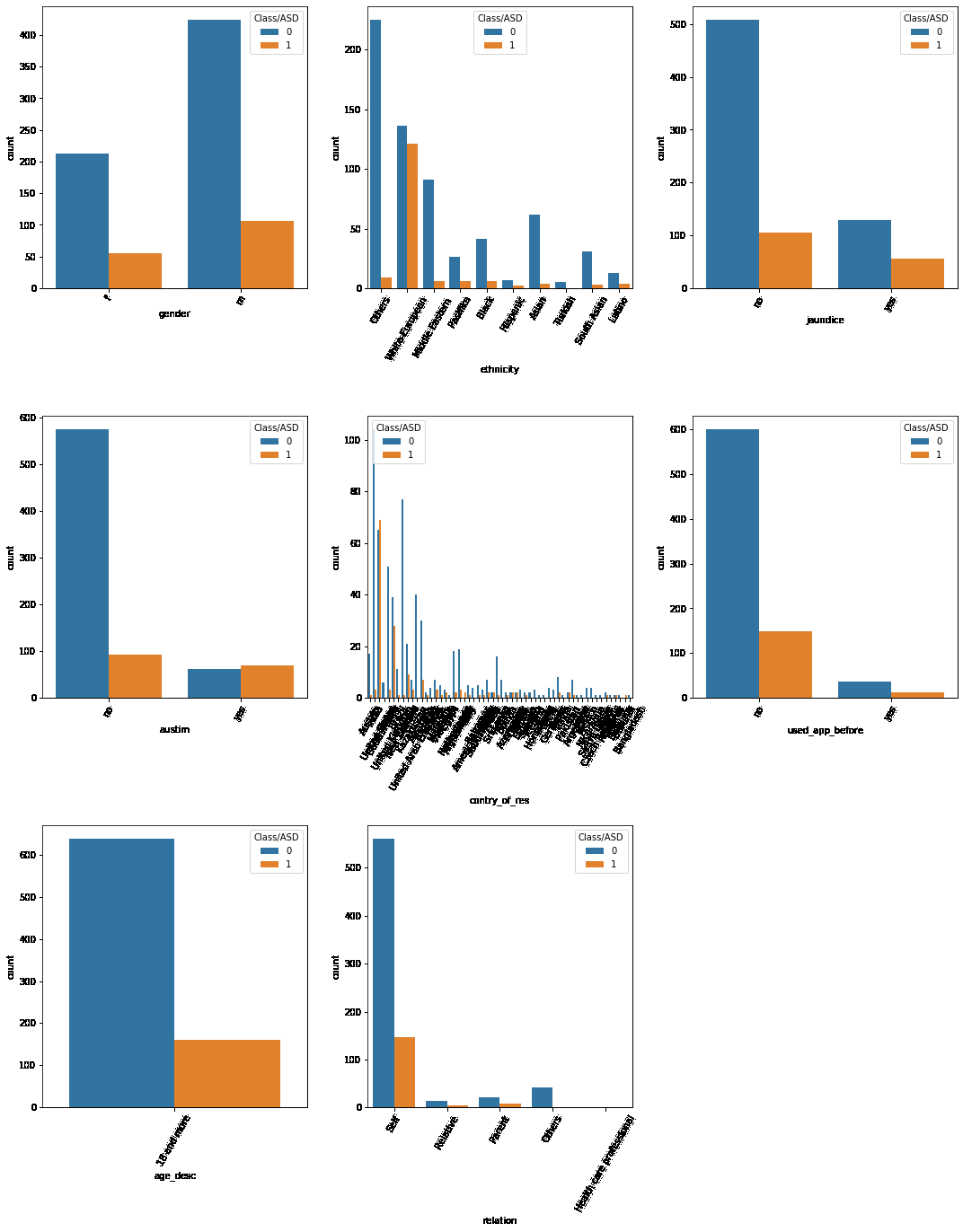Count plots for the categorical columns

From the above plots we can draw the following observations:

• Age_desc is the same for all the data points.
• This used_app_before feature doesn’t seem to be useful or it will be the source of data leakage.
• Here it seems like the chances of a male having autism is higher than a female but that is not true because we don’t have an equal number of examples of males and females.

## Python3

 `plt.figure(figsize``=``(``15``,``5``))``sb.countplot(data``=``df, x``=``'country_of_res'``, hue``=``'Class/ASD'``)``plt.xticks(rotation``=``90``)``plt.show()`

Output: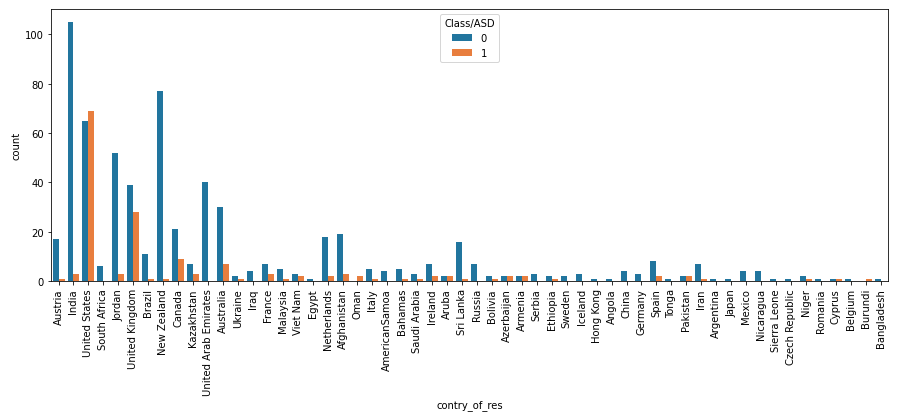Count plots for different country’s given in the dataset

In some places approximately 50% of the data available for that country have autism and in some places, this is quite low. This implies that the geography of a person also gives an idea of having autism.

## Python3

 `plt.subplots(figsize``=``(``15``,``5``))` `for` `i, col ``in` `enumerate``(floats):``  ``plt.subplot(``1``,``2``,i``+``1``)``  ``sb.distplot(df[col])``plt.tight_layout()``plt.show()`

Output: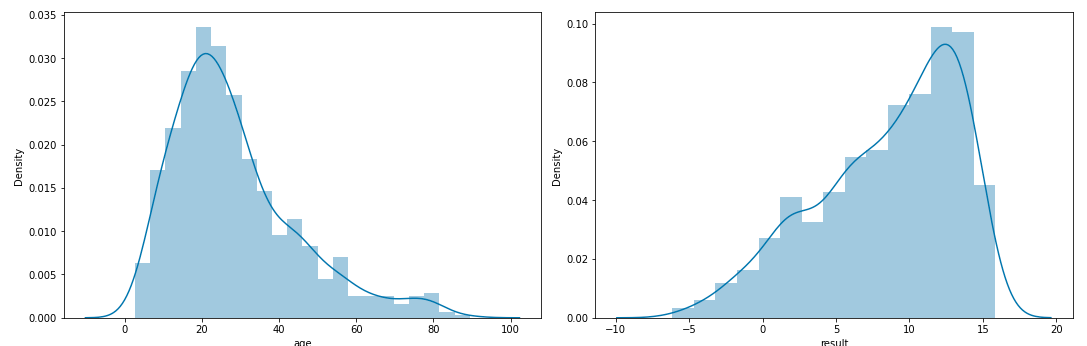Distribution plot for the columns with continuous data

Both of the continuous data are skewed left one is positive and the right one is negatively skewed.

## Python3

 `plt.subplots(figsize``=``(``15``,``5``))` `for` `i, col ``in` `enumerate``(floats):``  ``plt.subplot(``1``,``2``,i``+``1``)``  ``sb.boxplot(df[col])``plt.tight_layout()``plt.show()`

Output: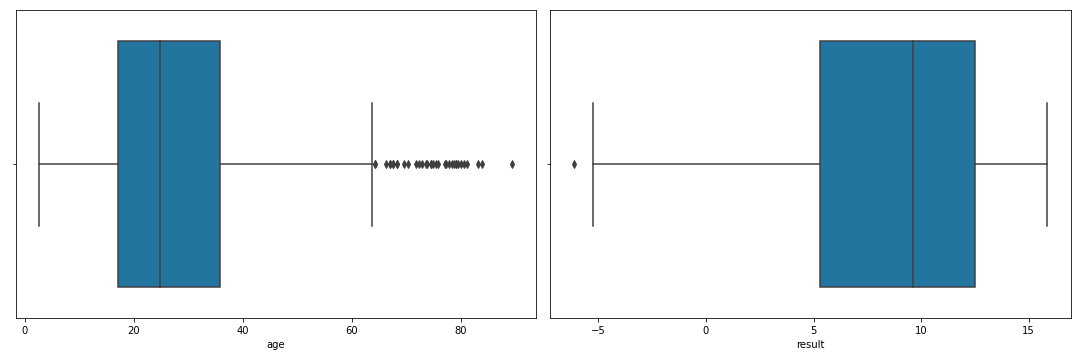Box plots for the columns with continuous data

Ah! some outliers in the result column. Let’s remove that as it doesn’t seem like too much loss of information.

## Python3

 `df ``=` `df[df[``'result'``]>``-``5``]``df.shape`

Output:

`(798, 22)`

So, here we lost only two data points.

## Feature Engineering

Feature Engineering helps to derive some valuable features from the existing ones. These extra features sometimes help in increasing the performance of the model significantly and certainly help to gain deeper insights into the data.

## Python3

 `# This functions make groups by taking``# the age as a parameter``def` `convertAge(age):``    ``if` `age < ``4``:``        ``return` `'Toddler'``    ``elif` `age < ``12``:``        ``return` `'Kid'``    ``elif` `age < ``18``:``        ``return` `'Teenager'``    ``elif` `age < ``40``:``        ``return` `'Young'``    ``else``:``        ``return` `'Senior'` `df[``'ageGroup'``] ``=` `df[``'age'``].``apply``(convertAge)`

Now, let’s explore the number of cases for each age group.

## Python3

 `sb.countplot(x``=``df[``'ageGroup'``], hue``=``df[``'Class/ASD'``])``plt.show()`

Output: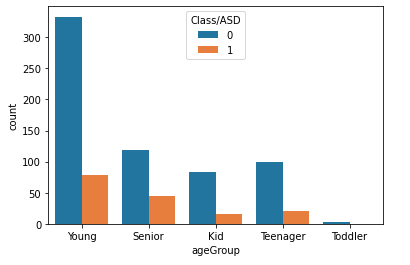Here we can conclude that the Young and Toddler group of people have lower chances of having Autism.

## Python3

 `def` `add_feature(data):``  ` `  ``# Creating a column with all values zero``  ``data[``'sum_score'``] ``=` `0``  ``for` `col ``in` `data.loc[:,``'A1_Score'``:``'A10_Score'``].columns:``    ` `    ``# Updating the 'sum_score' value with scores``    ``# from A1 to A10``    ``data[``'sum_score'``] ``+``=` `data[col]` `  ``# Creating a random data using the below three columns``  ``data[``'ind'``] ``=` `data[``'austim'``] ``+` `data[``'used_app_before'``] ``+` `data[``'jaundice'``]` `  ``return` `data` `df ``=` `add_feature(df)`

In the above code block, we have just summed up the clinical scores given from A1 to A10.

## Python3

 `sb.countplot(x``=``df[``'sum_score'``], hue``=``df[``'Class/ASD'``])``plt.show()`

Output: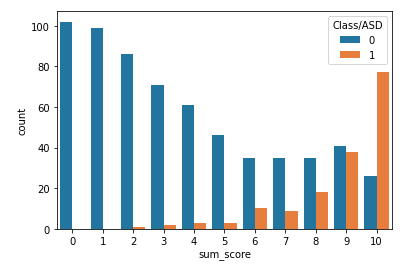Comparison between scores and number of positive and negative cases

Another amazing observation higher the sum score higher the chances of having autism is higher as well and similarly for lower sum scores that are for less than 5 it is rare that the person has autism.

## Python3

 `# Applying log transformations to remove the skewness of the data.``df[``'age'``] ``=` `df[``'age'``].``apply``(``lambda` `x: np.log(x))`

The age data given to us is positively skewed. As we know that skewed data impacts the performance of the model, we will apply log transformations to remove the skewness of the data.

## Python3

 `sb.distplot(df[``'age'``])``plt.show()`

Output: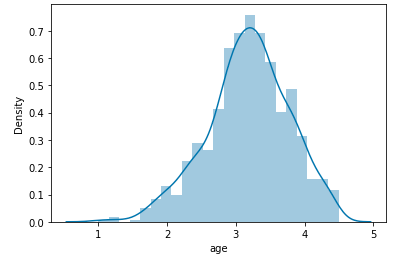Normal distribution of the age values after log transformations

Now, the skewness of the data has been removed successfully by using the log transformations.

## Python3

 `def` `encode_labels(data):``    ``for` `col ``in` `data.columns:``      ` `      ``# Here we will check if datatype``      ``# is object then we will encode it``      ``if` `data[col].dtype ``=``=` `'object'``:``        ``le ``=` `LabelEncoder()``        ``data[col] ``=` `le.fit_transform(data[col])``    ` `    ``return` `data` `df ``=` `encode_labels(df)` `# Making a heatmap to visualize the correlation matrix``plt.figure(figsize``=``(``10``,``10``))``sb.heatmap(df.corr() > ``0.8``, annot``=``True``, cbar``=``False``)``plt.show()`

Output: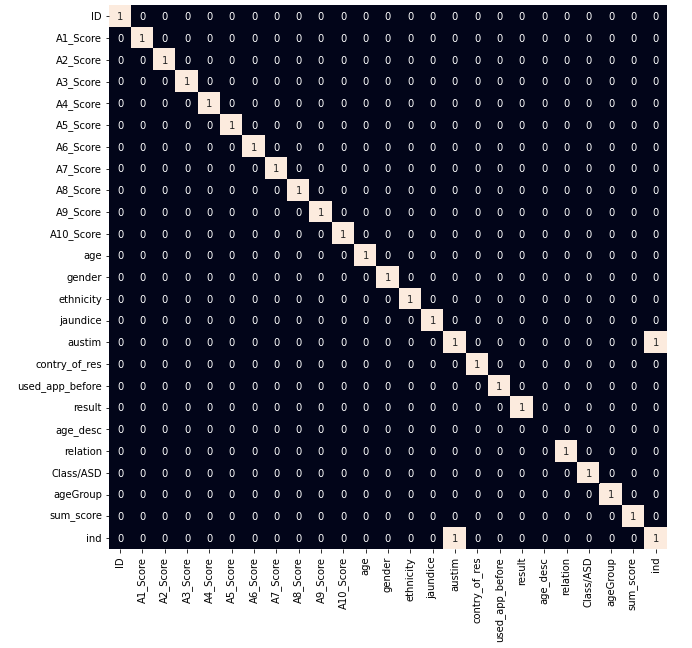Heat map for the highly correlated features

From the above heat map, we can see that there are only one highly correlated features which we will remove before training the model on this data as highly correlated features do not help in learning useful patterns in the data.

## Model Training

Now we will separate the features and target variables and split them into training and the testing data by using which we will select the model which is performing best on the validation data.

## Python3

 `removal ``=` `[``'ID'``, ``'age_desc'``, ``'used_app_before'``, ``'austim'``]``features ``=` `df.drop(removal ``+` `[``'Class/ASD'``], axis``=``1``)``target ``=` `df[``'Class/ASD'``]`

Let’s split the data into training and validation data. Also, the data was imbalanced earlier now we will balance it using the Random Over Sampler in this method we sample some points from the minority class and repeat it multiple times so, that the two classes get balanced.

## Python3

 `X_train, X_val, Y_train, Y_val ``=` `train_test_split(features, target, test_size ``=` `0.2``, random_state``=``10``)` `# As the data was highly imbalanced we will balance it by adding repetitive rows of minority class.``ros ``=` `RandomOverSampler(sampling_strategy``=``'minority'``,random_state``=``0``)``X, Y ``=` `ros.fit_resample(X_train,Y_train)``X.shape, Y.shape`

Output:

`((1026, 20), (1026,))`

Now, let’s normalize the data to obtain stable and fast training.

## Python3

 `# Normalizing the features for stable and fast training.``scaler ``=` `StandardScaler()``X ``=` `scaler.fit_transform(X)``X_val ``=` `scaler.transform(X_val)`

Now let’s train some state-of-the-art machine learning models and compare them which fit better with our data.

## Python3

 `models ``=` `[LogisticRegression(), XGBClassifier(), SVC(kernel``=``'rbf'``)]` `for` `model ``in` `models:``  ``model.fit(X, Y)` `  ``print``(f``'{model} : '``)``  ``print``(``'Training Accuracy : '``, metrics.roc_auc_score(Y, model.predict(X)))``  ``print``(``'Validation Accuracy : '``, metrics.roc_auc_score(Y_val, model.predict(X_val)))``  ``print``()`

Output: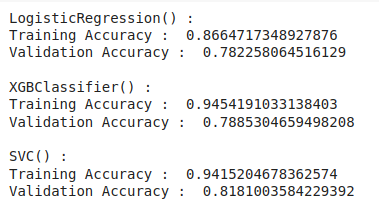Different state-of-the-art model accuracy on the test data

## Model Evaluation

From the above accuracies, we can say that Logistic Regression and SVC() classifier perform better on the validation data with less difference between the validation and training data. Let’s plot the confusion matrix as well for the validation data using the Logistic Regression model.

## Python3

 `metrics.plot_confusion_matrix(models[``0``], X_val, Y_val)``plt.show()`

Output: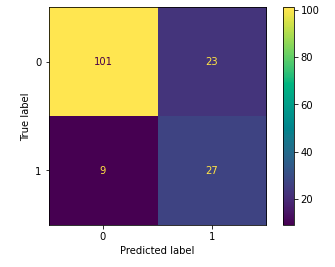Confusion matrix for the validation data

## Conclusion:

The machine learning model we have created is around 80% to 85% accurate. The disease for which there are no diagnostics methods machine learning models are able to predict whether the person has Autism or not. This is the power of machine learning by using which many of the real-world problems are being solved.

My Personal Notes arrow_drop_up